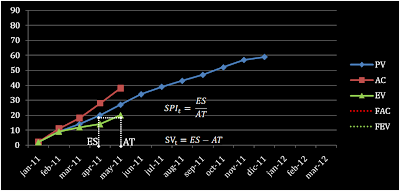## Monday, August 1, 2011

### EVM Forecasting Analysis

It's good reviewing graphically EVM forecasting in order to understand possibilities and limitations. The EVM most known formulas need certain corrections to be used in practical applications. These corrections came from the practice of "earned schedule". Let's remember the definition of Earned Schedule (ES), projecting the current value of EV over the PV curve. We could use the "t" following the acronym to differentiate the Schedule Performance Index calculated with ES from the classical Schedule Performance Index calculated as EV/PV, as you can see at following picture.Click to maximize

Using triangles similarities, it’s possible to obtain the Estimate At Completion (EAC) in the time dimension, as you can see at following picture.Click to maximize

Now we can remember that the maximum value of EV is BAC. Then we have an end point and with it we can trace a linear forecasting of EV.Click to maximize

Analogically, Cost Performance Index can be used as a ratio to forecast the Estimate At Completion in the dimension of cost. Using EAC it's possible to have a forecasting for AC.Click to maximize

This way, we can have a forecast for cost and time. This way of forecasting EV and AC is taking the past as enough information to predict the future. A better result is obtained if we categorize activities such way that the past performance is applied only to similar activities in the future.